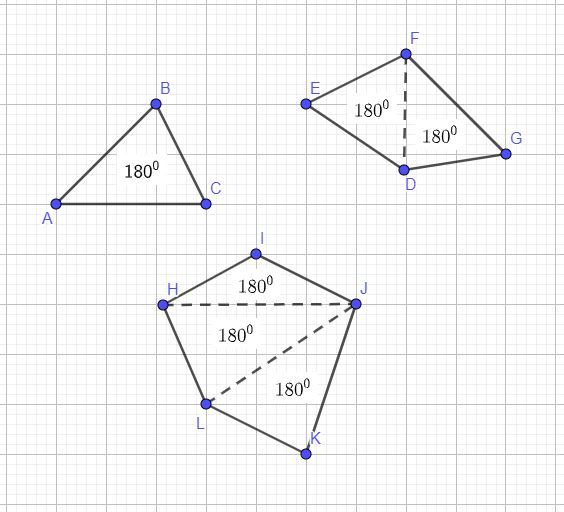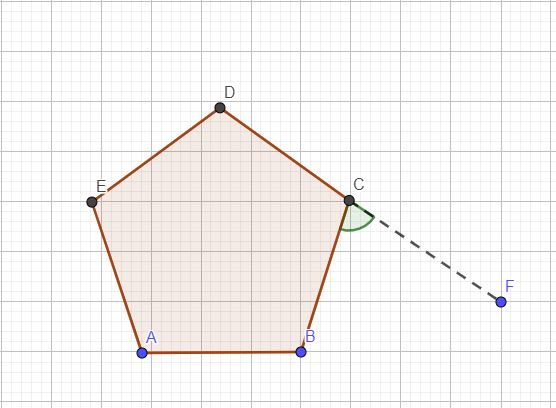# Mathematics

### Polygons

A polygon is a closed shape with many sides.

E.g. triangle, square, rhombus, decagon

In this tutorial, you are going to see how the interior angles and exterior angles vary in polygons, depending on the number of sides. You can choose the number of sides of a polygon and see how the interior and exterior angles change.

#### The Sum of Interior Angles

The sum of interior angles of a polygon, T, is given by,
T = (n-2)(180, where n is the number of sides.

Based on the following image, you can see that there is a connection between the number of triangles in a polygon and the sum of interior angles of the polygon.PolygonNo of SidesNo of Triangles InsideSum of Interior Angles
Triangle311 x 180
Square422 x 180
Pentagon533 x 180
Hexagon644 x 180
Heptagon755 x 180
Octagon866 x 180
Nonagon977 x 180
Decagon1088 x 180

If the sides of a polygon are equal, it is called a regular polygon. In a regular polygon, the interior angles are equal.

#### The Sum of Exterior Angles

The angle between a side and the the extended adjacent side is called exterior angle.The sum of exterior angles add up to 3600. For a regular polygon of sides n,
Exterior angle = 360 / number of sides

#### Polygon Maker - interactive practice

With the following applet, you can practise interior and exterior angles of a polygon.Maths is challenging; so is finding the right book. K A Stroud, in this book, cleverly managed to make all the major topics crystal clear with plenty of examples; popularity of the book speak for itself - 7th edition in print.

### Recommended - GCSE & iGCSEThis is the best book available for the new GCSE(9-1) specification and iGCSE: there are plenty of worked examples; a really good collection of problems for practising; every single topic is adequately covered; the topics are organized in a logical order.

### Recommended for A LevelThis is the best book that can be recommended for the new A Level - Edexcel board: it covers every single topic in detail;lots of worked examples; ample problems for practising; beautifully and clearly presented.